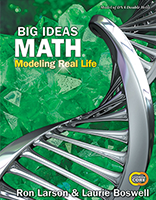1 Chapter 1 Numerical Expressions and Factors Section 1.1:  Powers and Exponents Also consider: Section 1.1:  Powers and Exponents Also consider: Section 1.2:  Order of Operations Also consider: Section 1.2:  Order of Operations Also consider: Section 1.3:  Prime Factorization Section 1.3:  Prime Factorization Section 1.4:  Greatest Common Factor Section 1.4:  Greatest Common Factor Section 1.5:  Least Common Multiple Section 1.5:  Least Common Multiple
 2 Chapter 2 Fractions and Decimals Section 2.1:  Multiplying Fractions Also consider: Section 2.1:  Multiplying Fractions Also consider: Section 2.2:  Dividing Fractions Also consider: Section 2.2:  Dividing Fractions Also consider: Section 2.3:  Dividing Mixed Numbers Also consider: Section 2.3:  Dividing Mixed Numbers Also consider: Section 2.4:  Adding and Subtracting Decimals Also consider: Section 2.4:  Adding and Subtracting Decimals Also consider: Section 2.5:  Multiplying Decimals Also consider: Section 2.5:  Multiplying Decimals Also consider: Section 2.6:  Dividing Whole Numbers Also consider: Section 2.6:  Dividing Whole Numbers Also consider: Section 2.7:  Dividing Decimals Also consider: Section 2.7:  Dividing Decimals Also consider:
 3 Chapter 3 Ratios and Rates Section 3.1:  Ratios Also consider: Section 3.1:  Ratios Also consider: Section 3.2:  Using Tape Diagrams Section 3.2:  Using Tape Diagrams Section 3.3:  Using Ratio Tables Section 3.3:  Using Ratio Tables Section 3.4:  Graphing Ratio Relationships Also consider: Section 3.4:  Graphing Ratio Relationships Also consider: Section 3.5:  Rates and Unit Rates Also consider: Section 3.5:  Rates and Unit Rates Also consider: Section 3.6:  Converting Measures Also consider: Section 3.6:  Converting Measures Also consider:
 4 Chapter 4 Percents Section 4.1:  Percents and Fractions Also consider: Section 4.1:  Percents and Fractions Also consider: Section 4.2:  Percents and Decimals Also consider: Section 4.2:  Percents and Decimals Also consider: Section 4.3:  Comparing and Ordering Fractions, Decimals, and Percents Section 4.3:  Comparing and Ordering Fractions, Decimals, and Percents Section 4.4:  Solving Percent Problems Section 4.4:  Solving Percent Problems
 5 Chapter 5 Algebraic Expressions and Properties Section 5.1:  Algebraic Expressions Also consider: Section 5.1:  Algebraic Expressions Also consider: Section 5.2:  Writing Expressions Also consider: Section 5.2:  Writing Expressions Also consider: Section 5.3:  Properties of Addition and Multiplication Section 5.3:  Properties of Addition and Multiplication Section 5.4:  The Distributive Property Also consider: Section 5.4:  The Distributive Property Also consider: Section 5.5:  Factoring Expressions Also consider: Section 5.5:  Factoring Expressions Also consider:
 6 Chapter 6 Equations Section 6.1:  Writing Equations in One Variable Also consider: Section 6.1:  Writing Equations in One Variable Also consider: Section 6.2:  Solving Equations Using Addition or Subtraction Also consider: Section 6.2:  Solving Equations Using Addition or Subtraction Also consider: Section 6.3:  Solving Equations Using Multiplication or Division Also consider: Section 6.3:  Solving Equations Using Multiplication or Division Also consider: Section 6.4:  Writing Equations in Two Variables Also consider: Section 6.4:  Writing Equations in Two Variables Also consider:
 7 Chapter 7 Area, Surface Area and Volume Section 7.1:  Areas of Parallelograms Also consider: Section 7.1:  Areas of Parallelograms Also consider: Section 7.2:  Areas of Triangles Also consider: Section 7.2:  Areas of Triangles Also consider: Section 7.3:  Areas of Trapezoids and Kites Also consider: Section 7.3:  Areas of Trapezoids and Kites Also consider: Section 7.4:  Three-Dimensional Figures Also consider: Section 7.4:  Three-Dimensional Figures Also consider: Section 7.5:  Surface Areas of Prisms Also consider: Section 7.5:  Surface Areas of Prisms Also consider: Section 7.6:  Surface Areas of Pyramids Also consider: Section 7.6:  Surface Areas of Pyramids Also consider: Section 7.7:  Volumes of Rectangular Prisms Section 7.7:  Volumes of Rectangular Prisms
 8 Chapter 8 Integers, Number Lines, and the Coordinate Plane Section 8.1:  Integers Also consider: Section 8.1:  Integers Also consider: Section 8.2:  Comparing and Ordering Integers Section 8.2:  Comparing and Ordering Integers Section 8.3:  Rational Numbers Also consider: Section 8.3:  Rational Numbers Also consider: Section 8.4:  Absolute Value Also consider: Section 8.4:  Absolute Value Also consider: Section 8.5:  The Coordinate Plane Also consider: Section 8.5:  The Coordinate Plane Also consider: Section 8.6:  Polygons in the Coordinate Plane Also consider: Section 8.6:  Polygons in the Coordinate Plane Also consider: Section 8.7:  Writing and Graphing Inequalities Also consider: Section 8.7:  Writing and Graphing Inequalities Also consider: Section 8.8:  Solving Inequalities Section 8.8:  Solving Inequalities
 9 Chapter 9 Statistical Measures Section 9.1:  Introduction to Statistics Section 9.1:  Introduction to Statistics Section 9.2:  Mean Section 9.2:  Mean Section 9.3:  Measures of Center Section 9.3:  Measures of Center Section 9.4:  Measures of Variation Section 9.4:  Measures of Variation Section 9.5:  Mean Absolute Deviation Also consider: Section 9.5:  Mean Absolute Deviation Also consider:
 10 Chapter 10 Data Displays Section 10.1:  Stem-and-Leaf Plots Section 10.1:  Stem-and-Leaf Plots Section 10.2:  Histograms Also consider: Section 10.2:  Histograms Also consider: Section 10.3:  Shapes of Distributions Section 10.3:  Shapes of Distributions Section 10.4:  Choosing Appropriate Measures • Coming soon: Choose appropriate measures to describe distributions Section 10.4:  Choosing Appropriate Measures • Coming soon: Choose appropriate measures to describe distributions Section 10.5:  Box-and-Whisker Plots Section 10.5:  Box-and-Whisker Plots
This document includes the IXL® skill alignments to Big Ideas Learning's Big Ideas Math 2019 curriculum. IXL provides skill alignments as a service to teachers, students, and parents. The skill alignments are provided by IXL and are not affiliated with, sponsored by, reviewed, approved or endorsed by Big Ideas Learning or any other third party. IXL® and IXL Learning® are registered trademarks of IXL Learning, Inc. All other intellectual property rights (e.g., unregistered and registered trademarks and copyrights) are the property of their respective owners.